University Physics Volume 1

# Challenge Problems

University Physics Volume 1Challenge Problems

### Challenge Problems

75.

A horizontal force $F→F→$ is applied to a uniform sphere in direction exact toward the center of the sphere, as shown below. Find the magnitude of this force so that the sphere remains in static equilibrium. What is the frictional force of the incline on the sphere?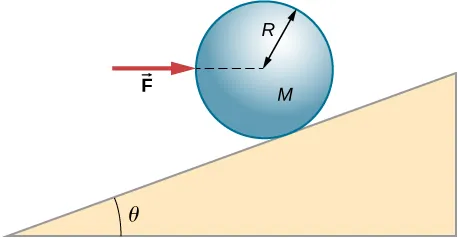76.

When a motor is set on a pivoted mount seen below, its weight can be used to maintain tension in the drive belt. When the motor is not running the tensions $T1T1$ and $T2T2$ are equal. The total mass of the platform and the motor is 100.0 kg, and the diameter of the drive belt pulley is $16.0cm.16.0cm.$ when the motor is off, find: (a) the tension in the belt, and (b) the force at the hinged platform support at point C. Assume that the center of mass of the motor plus platform is at the center of the motor.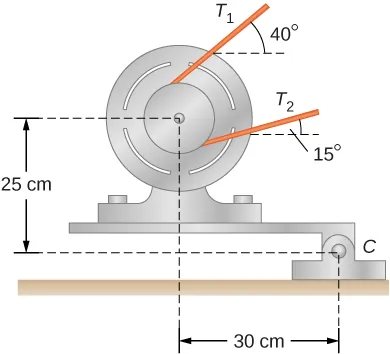77.

Two wheels A and B with weights w and 2w, respectively, are connected by a uniform rod with weight w/2, as shown below. The wheels are free to roll on the sloped surfaces. Determine the angle that the rod forms with the horizontal when the system is in equilibrium. Hint: There are five forces acting on the rod, which is two weights of the wheels, two normal reaction forces at points where the wheels make contacts with the wedge, and the weight of the rod.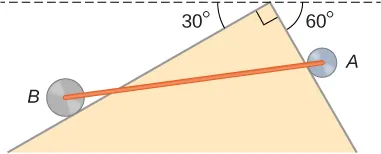78.

Weights are gradually added to a pan until a wheel of mass M and radius R is pulled over an obstacle of height d, as shown below. What is the minimum mass of the weights plus the pan needed to accomplish this?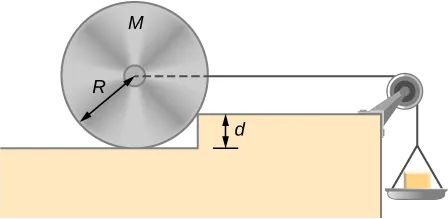79.

In order to lift a shovelful of dirt, a gardener pushes downward on the end of the shovel and pulls upward at distance $l2l2$ from the end, as shown below. The weight of the shovel is $mg→mg→$ and acts at the point of application of $F→2.F→2.$ Calculate the magnitudes of the forces $F→1F→1$ and $F→2F→2$ as functions of $l1,l1,$ $l2,l2,$ mg, and the weight W of the load. Why do your answers not depend on the angle $θθ$ that the shovel makes with the horizontal?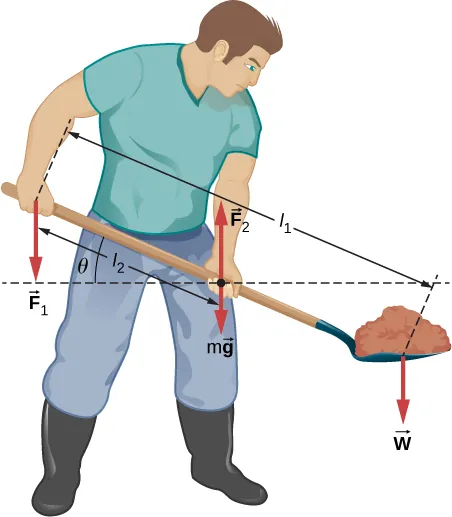80.

A uniform rod of length 2R and mass M is attached to a small collar C and rests on a cylindrical surface of radius R, as shown below. If the collar can slide without friction along the vertical guide, find the angle $θθ$ for which the rod is in static equilibrium.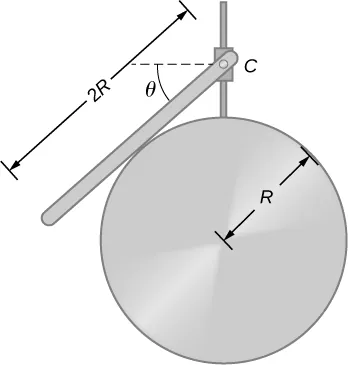81.

The pole shown below is at a $90.0°90.0°$ bend in a power line and is therefore subjected to more shear force than poles in straight parts of the line. The tension in each line is $4.00×104N,4.00×104N,$ at the angles shown. The pole is 15.0 m tall, has an 18.0 cm diameter, and can be considered to have half the strength of hardwood. (a) Calculate the compression of the pole. (b) Find how much it bends and in what direction. (c) Find the tension in a guy wire used to keep the pole straight if it is attached to the top of the pole at an angle of $30.0°30.0°$ with the vertical. The guy wire is in the opposite direction of the bend.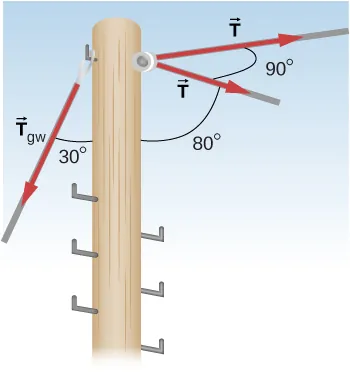Order a print copy

As an Amazon Associate we earn from qualifying purchases.